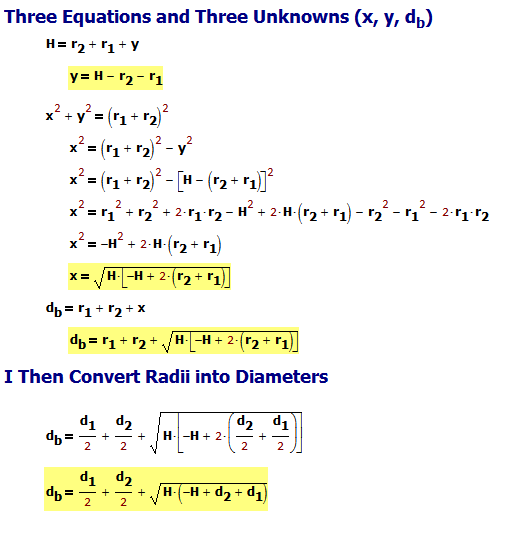# Bore Diameter Measurement Using Gage Balls

Quote of the Day

Cost is more important than quality, but quality is the best way to reduce cost.

Genichi Taguchi. I have much empirical evidence for the truth of his statement.

## Introduction

I am continuing to work through the metrology examples on this web page as part of junior machinist self-training. Today's technique shows how to use gage balls to measure the bore diameter of a cylinder (Figure 1). You can measure a bore diameter using a micrometer, but I have concerns that I might be measuring along a chord instead of a diameter – this error would result in too small of a result. The gage ball approach should eliminate that type of error.

In this post, I will work through the basic geometry associated with this measurement and will work an example.

## Background

I have discussed gage balls in two previous blog posts (here and here), which should provide any background that you need.

## Analysis

### Derivation

Figure 2 shows how I defined my variables. The analysis involves solving the right triangle formed by X, Y, and the line formed by r1 and r2.

Figure 3 shows the algebra involved with the solving for the bore diameter (dB). Because I work in Mathcad, most the algebraic manipulations are hidden. If you desire, you can see the algebra worked out in my response to a question below the post.

### Example

Figure 4 shows my results after applying the values in Figure 1 to the bore diameter formula. The result is very close to the bore diameter of 4.0000 on the scale drawing.

## Conclusion

I am almost done reviewing the use of roller gages and gage balls. A couple more  examples will complete my set of canonical applications.

Save

Save

Save

Save

Save

Save

Save

Save

Save

This entry was posted in Metrology. Bookmark the permalink.

### 4 Responses to Bore Diameter Measurement Using Gage Balls

1.Andrew says:

Thank you for taking the time to put together a most interesting and varied blog with the math theme. I see lots of interesting reading ahead. I found this page on a Google search for 'bore measurement using balls' and it answers my question exactly. Instead of just accepting the derived formula as correct, I have tried to follow the math, partly to blow the dust off 30 plus year high school algebra and to learn something new.

In following the math, I understand the 'given' part of figure 3 as well as the 'substitute' part but get completely lost on the 'eq1' part. The whole eq1 line makes no sense to me. When working Pythagoras with (r1+r2)^2 and y=(H-r2-r1) to solve for x, I end up with a negative expression which needs the square root taken to get x. From the 30 years back I recall that one cannot take a square root of a negative number.

Please can you enlighten me on how the algebra works in figure 3?

Regards
Andrew

•mathscinotes says:

Because I am lazy, I often let Mathcad simplify the formulas. You can manually solve them as shown below. Since x must be positive, I ignore the negative solution for x.mark

2.Andrew says:

Thank you for the explanation, it makes a lot more sense now.

Andrew

•mathscinotes says:

Don't hesitate to ask questions or make post requests. I am just a beginning machinist, but I have a fair amount of metrology experience.

mark